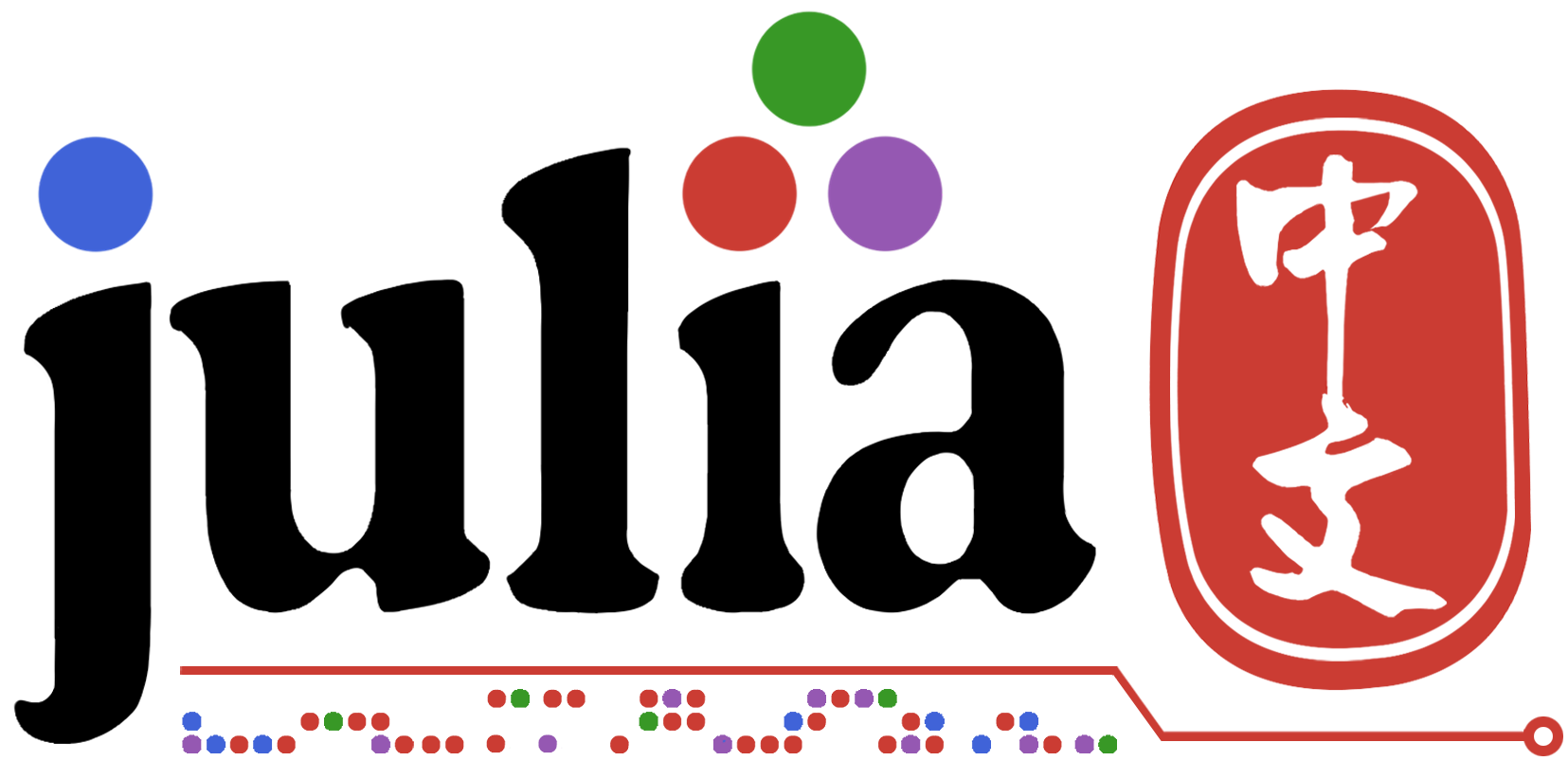An example for 官方文档-函数-默认值作用域

b=5
ff(x,a=b,b=1) = (a+b,x)
ff(3)

1 个赞
julia> ff(x,b=1,a=b) = (a+b,x)
ff (generic function with 3 methods)

julia> ff(3)
(2, 3)

b=5
ff(x,a=b,b=1)=(a+b,x)

a=b 的时候，后面的 b=1 还没有，因此用的就是外部的 b.

b=5
ff(x,b=1,a=b) = (a+b,x)

b=5,所以 a=1.

b=5
ff(x,b=1,a=b) = (a+b,x)

1 个赞

ff(x,b=1,a=b)=(a+b,x)

ff(x)
ff(x, b)
ff(x, b, a)

function ff(x, b, a)
(a+b, x)
end

function ff(x, b)
a = b
ff(x, b, a)
end

function ff(x)
b = 1
ff(x, b)
end

ff(x,a=b,b=1)=(a+b,x)

function ff(x, a, b)
(a+b, x)
end

function ff(x, a)
b = 1
ff(x, a, b)
end

function ff(x)
a = b
ff(x, a)
end

julia> ff(3)
ERROR: UndefVarError: b not defined